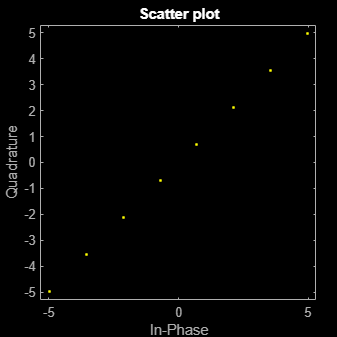Documentation

# pammod

Pulse amplitude modulation

## Syntax

```y = pammod(x,M) y = pammod(x,M,ini_phase) y = pammod(x,M,ini_phase,symbol_order) ```

## Description

`y = pammod(x,M)` outputs the complex envelope `y` of the modulation of the message signal `x` using pulse amplitude modulation. `M` is the alphabet size. The message signal must consist of integers between 0 and `M-1`. The modulated signal has a minimum Euclidean distance of 2. If `x` is a matrix with multiple rows, the function processes the columns independently.

`y = pammod(x,M,ini_phase)` specifies the initial phase of the modulated signal in radians.

`y = pammod(x,M,ini_phase,symbol_order)` specifies how the function assigns binary words to corresponding integers. If `symbol_order` is set to `'bin'` (default), the function uses a natural binary-coded ordering. If `symbol_order` is set to `'gray'`, it uses a Gray constellation ordering.

## Examples

collapse all

Generate random data symbols and apply pulse amplitude modulation.

Set the modulation order.

`M = 8;`

Generate random integers and apply PAM modulation having an initial phase of $\pi$/4.

```data = randi([0 M-1],100,1); modData = pammod(data,M,pi/4);```

Display the PAM constellation diagram.

`scatterplot(modData)`